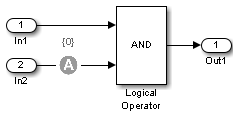# Proof Assumption

Constrain signal values when proving model properties

## Library

•## Description

When operating in property-proving mode, the Simulink® Design Verifier™ software proves that properties of your model satisfy specified criteria (see What Is Property Proving?). In this mode, you can use Proof Assumption blocks to define assumptions for signals in your model. The Values parameter lets you specify constraints on signal values during a property proof. The block applies the specified Values parameter to its input signal, and the Simulink Design Verifier software proves or disproves that the properties of your model satisfy the specified criteria.

The block's parameter dialog box also allows you to:

• Enable or disable the assumption.

• Specify that the block should display its Values parameter in the Simulink Editor.

• Specify that the block should display its output port.

### Note

The Simulink and Simulink Coder™ software ignore the Proof Assumption block during model simulation and code generation, respectively. The Simulink Design Verifier software uses the Proof Assumption block only when proving model properties.

### Specifying Proof Assumptions

Use the Values parameter to constrain signal values in property proofs. Specify any combination of scalars and intervals in the form of a MATLAB® cell array. For information about cell arrays, see Cell Arrays (MATLAB).

### Tip

If the Values parameter specifies only one scalar value, you do not need to enter it in the form of a MATLAB cell array.

Scalar values each comprise a single cell in the array, for example:

`{0, 5}`

A closed interval comprises a two-element vector as a cell in the array, where each element specifies an interval endpoint:

`{[1, 2]}`

Alternatively, you can specify scalar values using the `Sldv.Point` constructor, which accepts a single value as its argument. You can specify intervals using the `Sldv.Interval` constructor, which requires two input arguments, i.e., a lower bound and an upper bound for the interval. Optionally, you can provide one of the following values as a third input argument that specifies inclusion or exclusion of the interval endpoints:

• `'()'` — Defines an open interval.

• `'[]'` — Defines a closed interval.

• `'(]'` — Defines a left-open interval.

• `'[)'` — Defines a right-open interval.

### Note

By default, `Sldv.Interval` considers an interval to be closed if you omit its third input argument.

As an example, the Values parameter

`{0, [1, 3]}`

specifies:

• `0` — a scalar

• `[1, 3]` — a closed interval

The Values parameter

`{Sldv.Interval(0, 1, '[)'), Sldv.Point(1)}`

specifies:

• `Sldv.Interval(0, 1, '[)')` — the right-open interval [0, 1)

• `Sldv.Point(1)` — a scalar

If you specify multiple scalars and intervals for a Proof Assumption block, the Simulink Design Verifier software combines them using a logical OR operation during the property proof. In this case, the software considers the entire assumption to be satisfied if any single scalar or interval is satisfied.

## Data Type Support

The Proof Assumption block accepts signals of all built-in data types supported by the Simulink software. For a discussion on the data types supported by the Simulink software, see Data Types Supported by Simulink (Simulink). The block does not support complex input signals.

## Parameters

Enable

Specify whether the block is enabled. If selected (the default), the Simulink Design Verifier software uses the block when proving properties of a model. Clearing this option disables the block, that is, causes the Simulink Design Verifier software to behave as if the Proof Assumption block did not exist. If this option is not selected, the block appears grayed out in the Simulink Editor.

Type

Specify whether the block behaves as a Proof Assumption or Test Condition block. Select `Test Condition` to transform the Proof Assumption block into a Test Condition block.

Values

Specify the proof assumption (see Specifying Proof Assumptions).

Display values

Specify whether the block displays the contents of its Values parameter in the Simulink Editor. By default, this option is selected.

Pass through style (show Outport)

Specify whether the block displays an output port in the Simulink Editor. If selected (the default), the block displays its output port, allowing its input signal to pass through as the block output. If not selected, the block hides its output port and terminates the input signal. The following graphics illustrate the appearance of the block in each case.

Pass through style (show Outport): SelectedPass through style (show Outport): Deselected